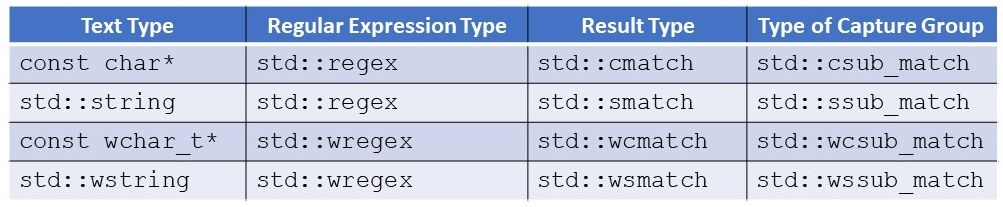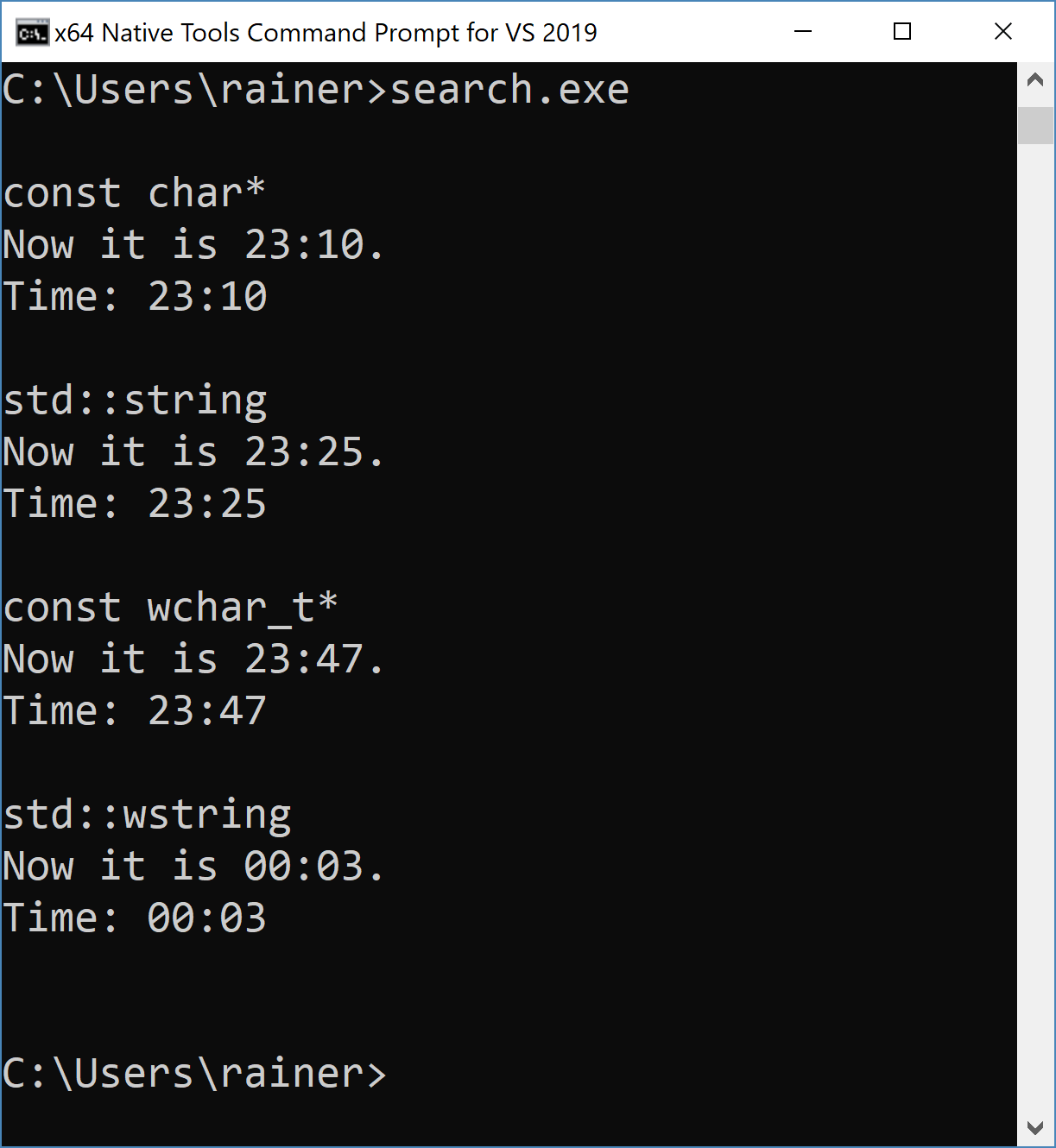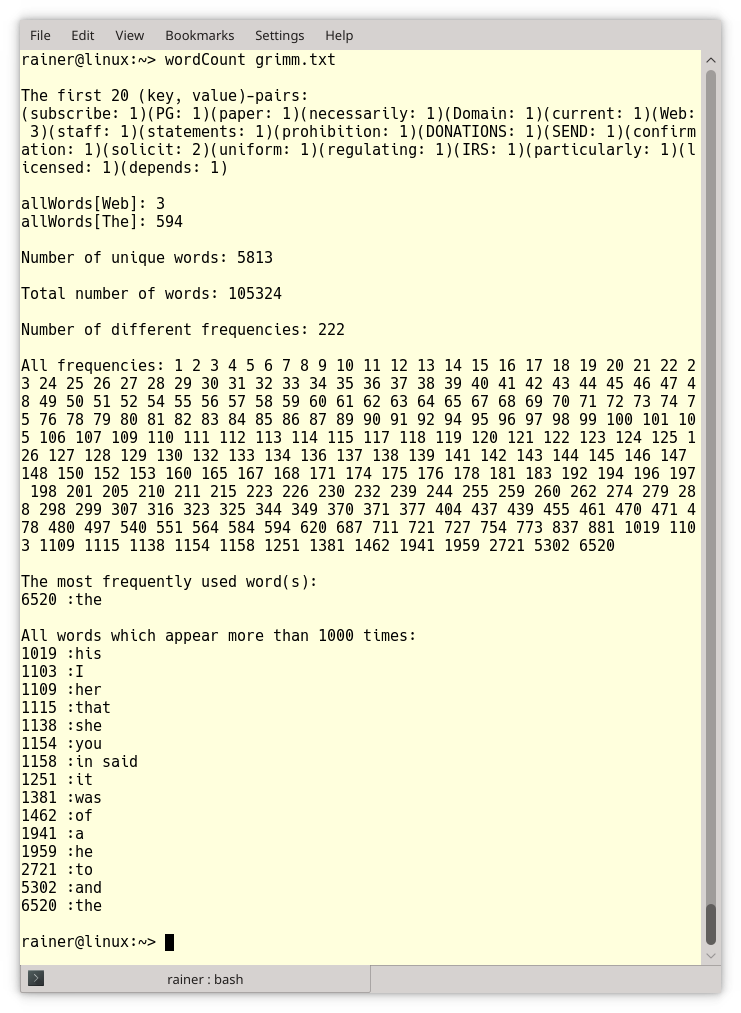# More Rules about the Regular Expression Library

There is more to write about the usage of regular expressions than I wrote in my last post The Regular Expression Library. Let’s continue.## The text determines the regular expression, the result, and the capture groups

First of all, the type of text determines the character type of the regular expression, the type of the search result, and the type of the capture group. Of course, my argument also holds if other parts of the regex machinery are applied to text. Okay, that sounds worse than it is. Capture is a subexpression in your search result, which you can define in round braces. I wrote already about it in my last post The Regular Expression Library.

The table gives all the types depending on the text type.##Modernes C++ Mentoring

Be part of my mentoring programs:

• "Fundamentals for C++ Professionals" (open)
• "Design Patterns and Architectural Patterns with C++" (open)
• "C++20: Get the Details" (reopens December 2023)
• Do you want to stay informed about my mentoring programs: Subscribe via E-Mail.

Here is an example of all the variations of std::regex_search depending on the text type.

```// search.cpp

#include <iostream>
#include <regex>
#include <string>

int main(){

std::cout << std::endl;

// regular expression for time
std::regex crgx("(?[0-9]|2[0-3]):[0-5][0-9]");

// const char*
std::cout << "const char*" << std::endl;
std::cmatch cmatch;

const char* ctime{"Now it is 23:10."};

if (std::regex_search(ctime, cmatch, crgx)){

std::cout << ctime << std::endl;
std::cout << "Time: " << cmatch << std::endl;

}

std::cout << std::endl;

// std::string
std::cout << "std::string" << std::endl;
std::smatch smatch;

std::string stime{"Now it is 23:25."};
if (std::regex_search(stime, smatch, crgx)){

std::cout << stime << std::endl;
std::cout << "Time: " << smatch << std::endl;

}

std::cout << std::endl;

// regular expression holder for time
std::wregex wrgx(L"(?[0-9]|2[0-3]):[0-5][0-9]");

// const wchar_t*
std::cout << "const wchar_t* " << std::endl;
std::wcmatch wcmatch;

const wchar_t* wctime{L"Now it is 23:47."};

if (std::regex_search(wctime, wcmatch, wrgx)){

std::wcout << wctime << std::endl;
std::wcout << "Time: " << wcmatch << std::endl;

}

std::cout << std::endl;

// std::wstring
std::cout << "std::wstring" << std::endl;
std::wsmatch wsmatch;

std::wstring  wstime{L"Now it is 00:03."};

if (std::regex_search(wstime, wsmatch, wrgx)){

std::wcout << wstime << std::endl;
std::wcout << "Time: " << wsmatch << std::endl;

}

std::cout << std::endl;

}
```

First, I used a const char*, a std::string, a const wchar_t*, and finally, a std::wstring as text. Because it is almost the same code in the four variations, from now on and for the rest of this post, I will only refer to the std::string.

The text contains a substring that stands for a time expression. Thanks to the regular expression (?[0-9]|2[0-3]):[0-5][0-9]“, I can search for it.  The regular expression defines a time format consisting of an hour and a minute, separated by a colon. Here is the hour and minute part:

• hour: ?[0-9]|2[0-3]:
• ?: 0 or 1 (optional)
• [0-9]: a number from 0 to 9
• |: stands for or
• 2[0-3]: 2 followed by a number from 0 to 3
• minute: [0-5][0-9]: a number from 0 to 5 followed by a number from 0 to 9

Finally, the output of the program.## Use regex_iterator or regex_token_iterator for repeated search

Don’t repeat std::search calls because you can quickly lose word boundaries or have empty hits. Use std::regex_iterator or std::regex_token_iterator for repeated search instead. std::regex_token_iterator allows you to address each capture group’s components or the text between the matches.

The “Hello World” of repeated search with regex is to count how often a word appears in a text. Here is the corresponding program.

```// wordCount.cpp

#include <algorithm>
#include <cstdlib>
#include <fstream>
#include <iostream>
#include <regex>
#include <string>
#include <map>
#include <unordered_map>
#include <utility>

using str2Int = std::unordered_map<std::string, std::size_t>;          // (1)
using intAndWords = std::pair<std::size_t, std::vector<std::string>>;
using int2Words= std::map<std::size_t,std::vector<std::string>>;

// count the frequency of each word
str2Int wordCount(const std::string &text) {
std::regex wordReg(R"(\w+)");                                        // (2)
std::sregex_iterator wordItBegin(text.begin(), text.end(), wordReg); // (3)
const std::sregex_iterator wordItEnd;
str2Int allWords;
for (; wordItBegin != wordItEnd; ++wordItBegin) {
++allWords[wordItBegin->str()];
}
return allWords;
}

// get to each frequency the words
int2Words frequencyOfWords(str2Int &wordCount) {
int2Words freq2Words;
for (auto wordIt : wordCount) {
auto freqWord = wordIt.second;
if (freq2Words.find(freqWord) == freq2Words.end()) {
freq2Words.insert(intAndWords(freqWord, {wordIt.first}));
} else {
freq2Words[freqWord].push_back(wordIt.first);
}
}
return freq2Words;
}

int main(int argc, char *argv[]) {

std::cout << std::endl;

// get the filename
std::string myFile;
if (argc == 2) {
myFile = {argv};
} else {
std::cerr << "Filename missing !" << std::endl;
exit(EXIT_FAILURE);
}

// open the file
std::ifstream file(myFile, std::ios::in);
if (!file) {
std::cerr << "Can't open file " + myFile + "!" << std::endl;
exit(EXIT_FAILURE);
}

std::stringstream buffer;
buffer << file.rdbuf();
std::string text(buffer.str());

// get the frequency of each word
auto allWords = wordCount(text);

std::cout << "The first 20 (key, value)-pairs: " << std::endl;
auto end = allWords.begin();
for (auto pair = allWords.begin(); pair != end; ++pair) {            // (4)
std::cout << "(" << pair->first << ": " << pair->second << ")";
}
std::cout << "\n\n";

std::cout << "allWords[Web]: " << allWords["Web"] << std::endl;      // (5)
std::cout << "allWords[The]: " << allWords["The"] << "\n\n";

std::cout << "Number of unique words: ";
std::cout << allWords.size() << "\n\n";                              // (6)

size_t sumWords = 0;
for (auto wordIt : allWords)
sumWords += wordIt.second;
std::cout << "Total number of words: " << sumWords << "\n\n";

auto allFreq = frequencyOfWords(allWords);

// (7)
std::cout << "Number of different frequencies: " << allFreq.size() << "\n\n";

std::cout << "All frequencies: ";                                    // (8)
for (auto freqIt : allFreq)
std::cout << freqIt.first << " ";
std::cout << "\n\n";

std::cout << "The most frequently used word(s): " << std::endl;      // (9)
auto atTheEnd = allFreq.rbegin();
std::cout << atTheEnd->first << " :";
for (auto word : atTheEnd->second)
std::cout << word << " ";
std::cout << "\n\n";

// (10)
std::cout << "All words which appear more than 1000 times:" << std::endl;
auto biggerIt =
std::find_if(allFreq.begin(), allFreq.end(),
[](intAndWords iAndW) { return iAndW.first > 1000; });
if (biggerIt == allFreq.end()) {
std::cerr << "No word appears more than 1000 times !" << std::endl;
exit(EXIT_FAILURE);
} else {
for (auto allFreqIt = biggerIt; allFreqIt != allFreq.end(); ++allFreqIt) {
std::cout << allFreqIt->first << " :";
for (auto word : allFreqIt->second)
std::cout << word << " ";
std::cout << std::endl;
}
}
std::cout << std::endl;
}
```

To better understand the program, I added a few comments to it.

The using declarations in line 1 help me to type less. The function wordCount determines the frequency of each word, and the function frequencyOfWords return to each frequency of all words. What is a word? Line 2 defines it with the regular expression, and line 3 uses it in a std::sregex_iterator. Let’s see which answers I can give with the two functions.

• Line 4: first 20 (key, value)-pairs
• Line 5: frequency of the words “Web” and “The”
• Line 6: number of unique words
• Line 7: number of frequencies
• Line 8: all appearing frequencies
• Line 9: the most frequently used word
• Line 10: words that appear more than 1000 times

Now, I need a lengthy text. Of course, I will use Grimm’s fairy tales from the project Gutenberg .  Here is the output:## What’s next?

I’m almost done with the regex functionality in C++, but I have one guideline in mind which makes repeated search often easier: Search not for the text patterns but the delimiters of the text patterns. I call this a negative search.

Thanks a lot to my Patreon Supporters: Matt Braun, Roman Postanciuc, Tobias Zindl, G Prvulovic, Reinhold Dröge, Abernitzke, Frank Grimm, Sakib, Broeserl, António Pina, Sergey Agafyin, Андрей Бурмистров, Jake, GS, Lawton Shoemake, Jozo Leko, John Breland, Venkat Nandam, Jose Francisco, Douglas Tinkham, Kuchlong Kuchlong, Robert Blanch, Truels Wissneth, Kris Kafka, Mario Luoni, Friedrich Huber, lennonli, Pramod Tikare Muralidhara, Peter Ware, Daniel Hufschläger, Alessandro Pezzato, Bob Perry, Satish Vangipuram, Andi Ireland, Richard Ohnemus, Michael Dunsky, Leo Goodstadt, John Wiederhirn, Yacob Cohen-Arazi, Florian Tischler, Robin Furness, Michael Young, Holger Detering, Bernd Mühlhaus, Matthieu Bolt, Stephen Kelley, Kyle Dean, Tusar Palauri, Dmitry Farberov, Juan Dent, George Liao, Daniel Ceperley, Jon T Hess, Stephen Totten, Wolfgang Fütterer, Matthias Grün, Phillip Diekmann, Ben Atakora, Ann Shatoff, Rob North, and Bhavith C Achar.

Thanks, in particular, to Jon Hess, Lakshman, Christian Wittenhorst, Sherhy Pyton, Dendi Suhubdy, Sudhakar Belagurusamy, Richard Sargeant, Rusty Fleming, John Nebel, Mipko, Alicja Kaminska, Slavko Radman, and David Poole.

 My special thanks to EmbarcaderoMy special thanks to PVS-StudioMy special thanks to Tipi.buildMy special thanks to Take Up Code## Seminars

I’m happy to give online seminars or face-to-face seminars worldwide. Please call me if you have any questions.

### Standard Seminars (English/German)

Here is a compilation of my standard seminars. These seminars are only meant to give you a first orientation.

• C++ – The Core Language
• C++ – The Standard Library
• C++ – Compact
• C++11 and C++14
• Concurrency with Modern C++
• Design Pattern and Architectural Pattern with C++
• Embedded Programming with Modern C++
• Generic Programming (Templates) with C++

#### New

• Clean Code with Modern C++
• C++20

### Contact Me

Modernes C++ Mentoring,Tags:
0 replies